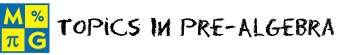# Pre-AlgebraThis page lists the Learning Objectives for all lessons in Unit 7.

#### Order of Operations

The student will be able to:

• Define arithmetic operations, fraction bar and vinculum.
• Restate the basic rules for order of operations.
• Recognize the need for such rules in mathematics.
• Evaluate arithmetic expressions by applying the rules.
• Apply order of operations rules to complete five interactive exercises.

#### Operations With Exponents

The student will be able to:

• Examine an arithmetic expression that includes an exponent.
• Restate the expanded rules for order of operations.
• Examine examples in which the expanded rules are applied to arithmetic expressions.
• Describe how to evaluate an arithmetic expression using the order of operations.
• Restate the PEMDAS mnemonic for the order of operations.
• Evaluate arithmetic expressions by applying the expanded rules.
• Apply the revised rules to complete five interactive exercises.

#### Operations With Integers

The student will be able to:

• Define integers.
• Review the order of operations and PEMDAS.
• Evaluate examples in which integers are included in arithmetic expressions.
• Describe the use of parentheses and brackets to decipher expressions involving integers.
• Examine examples in which fractions are included in arithmetic expressions.
• Describe how division applies to expressions that include fractions.
• Evaluate arithmetic expressions by applying the expanded rules.
• Summarize the complete set of rules for the order of operations, including brackets and fractions.
• Apply the expanded rules to complete five interactive exercises.

#### Algebraic Expressions

The student will be able to:

• Define variables, numerical expressions and algebraic expressions.
• Examine examples in which phrases are translated into algebraic expressions.
• Examine real-world problems in which phrases are translated into algebraic expressions.
• Explain the use of different letters to represent a variable.
• Translate phrases in algebraic expressions.
• Apply procedures to complete five interactive exercises.

#### Algebraic Equations

The student will be able to:

• Define algebraic equation.
• Examine examples in which open sentences are translated into algebraic equations.
• Examine real-world problems in which open sentences are translated into algebraic equations.
• Explain how different variables can be used to represent unknown quantities in the equations presented.
• Translate open sentences in algebraic equations.
• Apply procedures to complete five interactive exercises.

#### Practice Exercises

The student will be able to:

• Examine ten interactive exercises for all topics in this unit.
• Determine which rules and procedures are needed to complete each practice exercise.
• Compute answers by applying appropriate rules and procedures.
• Self-assess knowledge and skills acquired from this unit.

#### Challenge Exercises

The student will be able to:

• Evaluate ten interactive exercises with word problems for all topics in this unit.
• Analyze each word problem to identify the given information.
• Formulate a strategy for solving each problem.
• Apply strategies to solve routine and non-routine problems.
• Synthesize all information presented in this unit.
• Identify connections between pre-algebra and the real world.
• Develop problem-solving skills.

#### Solutions

The student will be able to:

• Examine the solution for each exercise presented in this unit.
• Identify which solutions need to be reviewed.
• Compare solutions to completed exercises.
• Identify and evaluate incorrect solutions.
• Repeat exercises that were incorrectly answered.
• Identify areas of strength and weakness.
• Decide which rules and procedures need to be reviewed from this unit.

### Order your CD today for Home or SchoolOR